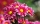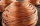# Length - math word problems

1. ContainerThe container-shaped box with internal dimensions of 3.9 m, 3.25 m and 2.6 m was completely filled with goods in the same cubic boxes. How long edge could this box have?
2. Proportion 2A car is able to travel 210 km in 3 hours. How far can it travel in 5 hours? Put what kind of proportion is this and show your solution.
3. Distance of linesFind the distance of lines AE, CG in cuboid ABCDEFGH, if given | AB | = 3cm, | AD | = 2 cm, | AE | = 4cm
4. After 2After we planted flowers in 2/5 of our garden, 24m remained unplanted. How many meters is the garden in total? If the total area of the garden is 1, the proportion of the remaining area is?
5. 3d printer3D printing ABS filament with diameter 1.75 mm has density 1.04 g/cm3. Find the length of m = 5 kg spool filament. (how to calculate length)
6. DecagonCalculate the area and circumference of the regular decagon when its radius of a circle circumscribing is R = 1m
7. Cyclist 9A cyclist travels at a a speed of 4.25 km per hour. At that rate, how far can he travel in 3.75 hours?
8. Wiring 2Willie cut a piece of wire that was 3/8 of the total length of the wire. He cut another piece that was 9m long. The two pieces together were one half of the total length of the wire. How long was the wire before he cut it?
9. RunningJoanne ran 8 1/2 Kyleigh 1 2/3 and John 6 3/4 miles in one day. How many miles did the girls run in 3 days?
10. RectanglesHow many different rectangles with sides integers (in mm) have a circumference exactly 1000 cm?
11. HectolitersHow many hectoliters of water is in garden barrel with 90 cm diameter and a height of 1.3 m, if it is filled to 80% of its capacity?
12. SandboxSandbox has area of 32 sq ft and length of 4 1/2 ft. What is width of sandbox.
13. Length subtractingExpress in mm: 5 3/10 cm - 2/5 mm
14. Christmas napkinsThe girls embroidered Christmas napkins. Each napkin had the shape of a triangle with sides of 5 dm, 60 cm, and 800 mm. How many cms did the girls sew if they made 15 napkins?
15. Sand pathHow many m3 of sand is needed to fill the 1.5m wide path around a rectangular flowerbed of 8m and 14m if the sand layer is 6cm high?
16. An oilAn oil drum is cut in half. One half is used as a water trough. Use the dimensions; length 82cm, width 56cm to estimate the capacity of the water trough in liters.
17. Area of iso-trapFind the area of an isosceles trapezoid if the lengths of its bases are 16 cm and 30 cm, and the diagonals are perpendicular to each other.
18. Big cubeCalculate the surface of the cube, which is composed of 64 small cubes with an edge 1 cm long.
19. Customary lengthConvert length 65yd 2 ft to ft
20. Find theFind the volume of a quadrangle prism high 2dm whose base is a square with a side 15cm.

Do you have an interesting mathematical word problem that you can't solve it? Submit math problem, and we can try to solve it.

We will send a solution to your e-mail address. Solved examples are also published here. Please enter the e-mail correctly and check whether you don't have a full mailbox.

Please do not submit problems from current active competitions such as Mathematical Olympiad, correspondence seminars etc...

Do you want to convert length units?